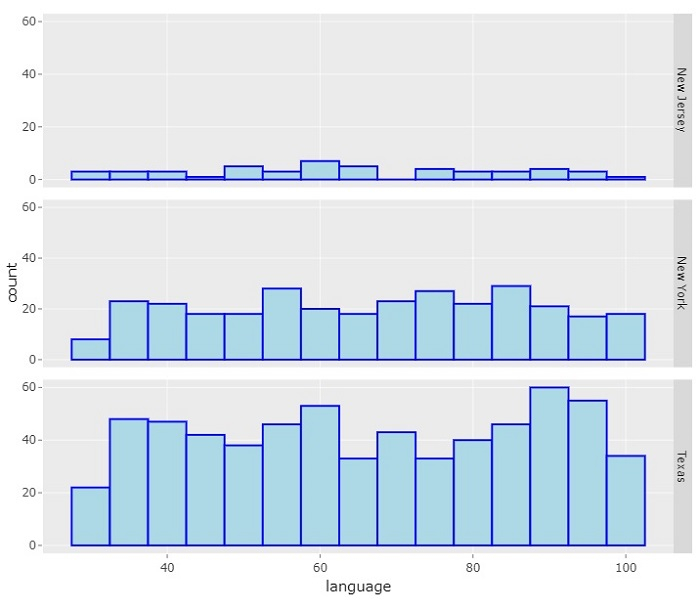# How to show multiple ggplot2 plots with Plotly using R?

R is a programming language for statistical computing and graphics. ggplotly() is a function used to convert static plots to web-based plots. ggplotly() returns a Plotly object. In this tutorial, we will see how to show multiple ggplot2 plots with Plotly using R.

• Here, we will use the aes() function that is used for aesthetic mapping between visual cue and a variable. It contains the following arguments: position (X and Y axes), color, fill, shape, line type, and size.

• To display multiple ggplot2 plots, we will use the facet_grid() function.

Follow the steps given below to show multiple ggplot2 plots with R language.

Step 1

Make sure you have the following libraries installed

install.packages('ggplot2')
install.packages('plotly')
install.packages("ggpubr")


Step 2

After installing, import these libraries −

library(readr)
library(ggplot2)
library(plotly)
library("ggpubr")


Step 3

Create a dataset from an external CSV file. Here, we have imported the "students_result.csv" file. You need to keep the CSV file in the project directory or provide its full path in the following command −

students_result <- read_csv("students_result.csv")


Step 4

Create a ggplot using the following arguments −

p1 = ggplot(students_data) + geom_histogram(mapping = aes(x=language), color='blue', fill='lightblue', bins=15)


Step 5

Use the facet_grid() method to create a figure with multiple ggplot2 plots

figure = p1 + facet_grid(rows = vars(state))


Step 6

Create a ggplot from the figure.

ggplotly(figure)


## Example

Here is the complete code to show multiple ggplot2 plots with Plotly using R −

install.packages('ggplot2')
install.packages('plotly')
install.packages("ggpubr")

library(ggplot2)
library(plotly)
library("ggpubr")

p1 = ggplot(students_data) + geom_histogram(mapping = aes(x=language), color='blue', fill='lightblue', bins=15)

figure = p1 + facet_grid(rows = vars(state))

ggplotly(figure)


## Output

It will produce the following output on the browser −